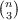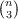#### Vol. 25, No. 2, 1968

 Download this articleFor screen For printingRecent Issues Vol. 325: 1  2 Vol. 324: 1  2 Vol. 323: 1  2 Vol. 322: 1  2 Vol. 321: 1  2 Vol. 320: 1  2 Vol. 319: 1  2 Vol. 318: 1  2Online Archive Volume: Issue:The Journal Subscriptions Editorial Board Officers Contacts Submission Guidelines Submission Form Policies for Authors ISSN: 1945-5844 (e-only) ISSN: 0030-8730 (print) Special Issues Author Index To Appear Other MSP Journals
On the tetrahedral graph

### Martin Aigner

Vol. 25 (1968), No. 2, 219–227
##### Abstract

Generalizing the conccpt of the triangular association scheme, Bose and Laskar introduced the tetrahedral graph the vertices of which are theunordered triplets selected from n symbols with two points adjacent if and only if their corresponding triplets have two symbols in common. If we let d(x,y) denote the distance between two vertices x, y and Δ(x,y) the number of vertices adjacent to both x and y, then the tetrahedral graph possesses the following 4 properties:

(B0) the number of vertices is(B1) it is connected and regular of degree 3(n 3)

(B2) if d(x,y) = 1 then Δ(x,y) = n 2

(B3) if d(x,y) = 2 then Δ(x,y) = 4.

The question whether these conditions characterize tetrahedral graphs (no loops or parallel edges permitted) was answered in the affirmative by Bose and Laskar for n > 16. In the present paper characterizations of tetrahedral graphs are derived by strengthening each one of (B1), (B2), (B3) and these results are utilized to prove the sufficiency of (B0)–(B3) for n = 6. (For n < 4 the problem is void, n = 4,5 are trivial cases.)

Primary: 05.40
##### Milestones